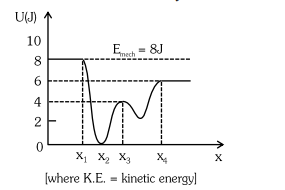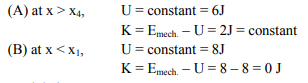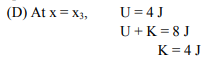# Given below is the plot of a potential energy function U(x) for a system, in which a particle is in one dimensional motion,Question:

Given below is the plot of a potential energy function U(x) for a system, in which a particle is in one dimensional motion, while a conservative force F(x) acts on it. Suppose that Emech = 8 J, the incorrect statement for this system is :1. at $\mathrm{x}>\mathrm{x}_{4}, \mathrm{~K}$.E. is constant throughout the region.

2. at $x 3. at$x=x_{2}, K . E$. is greatest and the particle is moving at the fastest speed. 4. at$x=x_{3}, K . E,=4 J$. Correct Option: , 2 Solution:$\mathrm{E}_{\text {mech. }}=8 \mathrm{~J}$Particle is at rest. (C) At$x=x_{2}, U=0 \Rightarrow E_{\text {mech. }}=K=8 \mathrm{~J}\$KE is greatest, and particle is moving at fastest speed.